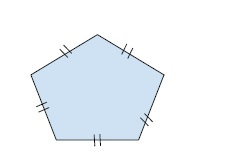# Program to find the Area of a Pentagon in C++

In this problem, we are given a number n that denotes that side of the pentagon. Our task is to create a program to find the Area of a Pentagon in C++.

Pentagon is a five-sided geometric figure.Regular pentagon is a pentagon with all five sides and angles equal.

Let’s take an example to understand the problem,

## Input

a = 7

## Output

84.3

Program to illustrate the working of our solution,

## Example

Live Demo

#include <iostream>
using namespace std;
float calcpentagonArea(int a){
return ( ((6.8819)*a*a)/4);
}
int main() {
int a = 7;
cout<<"The area of regular pentagon of side "<<a<<" is"<<calcpentagonArea(a);
return 0;
}

## Output

The area of regular pentagon of side 7 is 84.3033